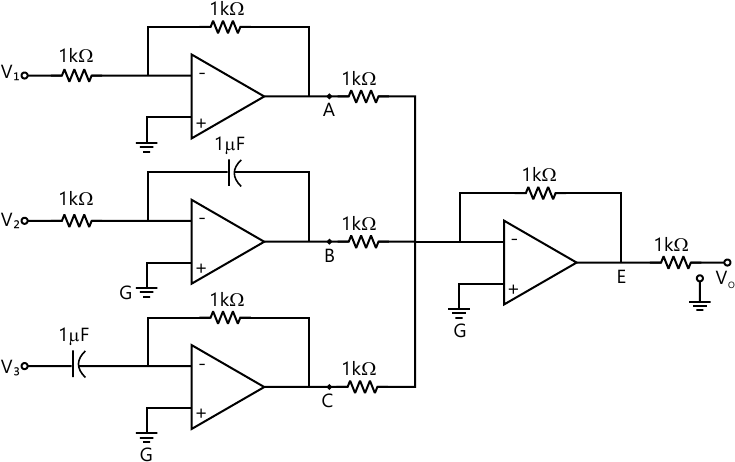MORE IN Basic Electronics
VTU First Year Engineering (C Cycle) (Semester 1)
Basic Electronics
December 2015
Total marks: --
Total time: --
INSTRUCTIONS
(1) Assume appropriate data and state your reasons
(2) Marks are given to the right of every question
(3) Draw neat diagrams wherever necessary

1 (a) Draw and explain the V-I characteristics of silicon P-N junction Diode.
5 M
1 (b) A single phase full-wave rectifire supplies power to a 1 KΩ load. The AC voltage applied to the diode is 300-0-300 V. If diode resistance is 25Ω and that of the transformer secondary negligible. Determine average load current, average load voltage and rectification efficiency.
6 M
1 (c) Draw and explain the series negative clipper circuit with a suitable waveform.
4 M
1 (d) Derive an equation for the collector current of a P-N transistor in terms of base current and αdc and also obtaina the relationship between αdc and βdc.
5 M

2 (a) With a neat circuit diagram and waveform, explain the working of a half-wave rectifier and show that its ripple factor is 1.21.
7 M
2 (b) Draw the common-emitter circuit and sketch the input and output characteristics. Also explain operating regions by indicating them on the characteristics curve.
7 M
2 (c) Explain the performance of Zener-Diode in terms of source and load effects.
6 M

3 (a) Explain the operation of a fixed, bias circuit with a suitable circuit diagram.
7 M
3 (b) What are the ideal characteristics of a operational amplifier?
5 M
3 (c) A voltage-divider bias circuit has VCC=15V, RC=2.7 KΩ and RC=2.2 KΩ, R1=22KΩ R3=12KΩ, bfc=50. Calculate VE, VC, IC and VCE and also draw DC load line and mark the Q point. Assume VBB=0.7V.
8 M

4 (a) Derive the expression of output voltage of a differentiator circuit using an opamp.
5 M
4 (b) Write expression for output voltage at points A, B, C, D and E as shown in Fig. 4(b).10 M
4 (c) With a neat circuit diagram and waveform, explain how opamp can be used as a non- inverting amplifier.
5 M

5 (a) State De-Morgan's theorem for 4- variable and prove them by the method of perect induction.
6 M
5 (b) Design full-adder circuit using three- variables and implement it using two half-adder.
6 M
5 (c) Explain the construction of an OR-gate using diodes.
4 M
5 (d) Realize basic gates from NAND gate.
4 M

6 (a) Design a logic circuit, symbol and truth-table of exclusive-OR gate.
4 M
6 (b) Convert:
i) (294.6875)10 - ()s
ii) (356.15)8 ()2- ()10
4 M
6 (c) Simplify and Realize using basic gates:
$i) \ ABC+ A\bar{B}C+ AB\bar{C}+ \bar{A}BC \\ii) \ (\overline{A+B}) (\bar{A}+ \bar{C}) (\bar{B}+ C)$
5 M
6 (d) Substract (111)1 from (1010)2 using 1's 2's complement method.
5 M

7 (a) Explain the working of clocked R-S flip flop with a suitable logic diagram and a truth table.
6 M
7 (b) Explain the logic pinout and signal of 8085 microprocessor.
8 M
7 (c) Explain the construction and working of a linear variable differential transducer[LVDT].
6 M

8 (a) With a neat block diagram, explain the architecture of 8051 microcontroller.
9 M
8 (b) What is transducer? Distinguish between active and passive transducer.
6 M
8 (c) What is Thermister? Explain its advantages and limitations.
5 M

9 (a) Define amplitude modulation and prove that $P_t= P_c \left ( 1+ \dfrac {m^2}{2} \right )$
5 M
9 (b) What are commonly used frequency ranges in communication system? Mention the application of each range.
5 M
9 (c) The total power content of an AM signal is 1000 W. Determine the power begin transmitted at carrier frequency and at each of the side bands when percentage modulation is 100%.
5 M
9 (d) Explain the differences between Amplitude and Frequency modulation.
5 M

10 (a) Explain the block diagram of ISDN.
6 M
10 (b) What are the advantages of optical fiber communication?
5 M
10 (c) Explain the basic principle of operation of mobile phones.
5 M
10 (d) What is modulation? Explain need of modulation.
4 M

More question papers from Basic Electronics